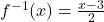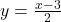## Which function is the inverse of f(x) = 2x+37 O О O ‘(x) –2x+3 Of(z) = 23+3 answer ?????

Question

Which function is the inverse of f(x) = 2x+37
O
О
O ‘(x) –2x+3
Of(z) = 23+3

in progress 0
6 months 2021-08-18T17:05:20+00:00 1 Answers 5 views 0Step-by-step explanation:

y = f(x)

y = 2x+3

You need to switch the places of x and y, into this:

x = 2y + 3

Then solve for y:

2y = x-3